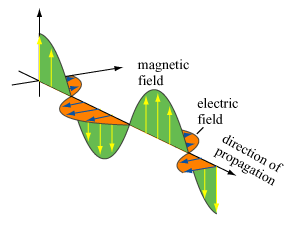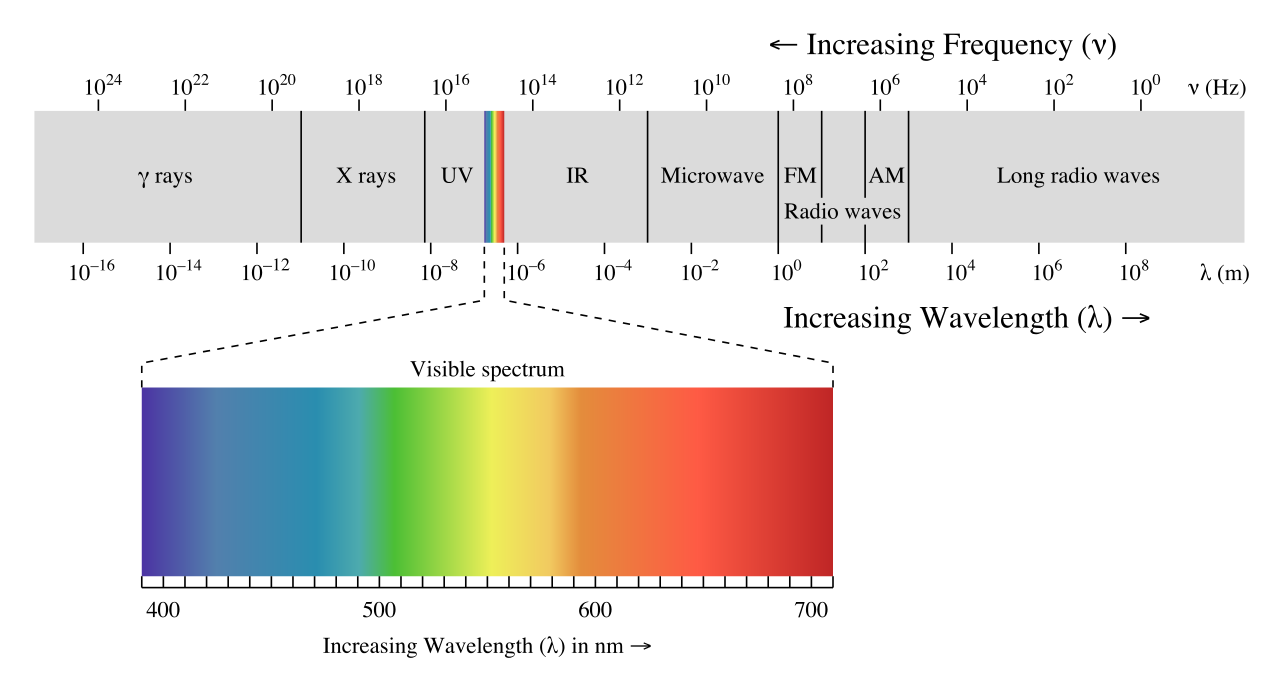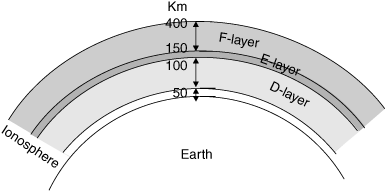Click to Chat

1800-1023-196

+91-120-4616500

CART 0

• 0

MY CART (5)

Use Coupon: CART20 and get 20% off on all online Study Material

ITEM
DETAILS
MRP
DISCOUNT
FINAL PRICE
Total Price: Rs.

There are no items in this cart.
Continue Shopping• Complete JEE Main/Advanced Course and Test Series
• OFFERED PRICE: Rs. 15,900
• View Details

Revision Notes on Electromagnetic WavesElectromagnetic wave

?Electric and magnetic fields fluctuating together can form a propagating wave, appropriately called an electromagnetic wave.

Equation of plane progressive electromagnetic wave

E = E0 sin ω (t – x/c)

B = B0 sin ω (t – x/c)

Here, ω = 2πf

Properties of elctromagnetic wave

(a) ?These waves are transverse in nature and it does not require a medium for propagation.

(b) These waves propagate through space with speed of light, i.e., 3108 m/s.

(c) It carries energy as it propagates. The higher the frequency, the higher the energy associated with the wave.

(d) It can transfer its energy to the matter on which it impinges.

(e) Its propagation obeys the inverse square law.

(f) It can be used to carry information.

(g) It can be be reflected or refracted.

(h) It can be split and recombined to form diffraction patterns.

(i) It can travel great distances. The radiation resulting from a simple100 volt, 1 MHz sine wave fed into a suitable antenna can be detected as far away as the next planet.

(j) It travels in straight lines.

(k) It can be bent around the Earth's circumference by reflection from the ionosphere.

(l) It can pass through walls.

(m) It can be captured by placing a metal rod, a loop, parabolic metal dish or horn in its path and it can be launched into the atmosphere with the same tools.

(n) The E and B fields are perpendicular to each other.

(o) The E and B fields are in phase (both reach a maximum and minimum at the same time).

(p) The E and B fields are perpendicular to the direction of travel (transverse waves).

Speed of electromagnetic wave

c = 1/√µ0ε0

Here, µ0 is permeability of free space and ε0 is the permitivity of free space.

c = E0/B0

Here E0 and B0 are maximum values of electric and magnetic field vector.

P = q2a2/6πε0c2

Poynting vector

The rate of flow of energy in an electromagnetic wave is described by the vector S called the poynting vector.

S = (1/µ0) [EB]

SI unit of S is watt/m2

The energy in electromagnetic waves is divided equally between electric field and magnetic field vectors.
Average electric energy density

UE = ½ ε0E2 = ¼ ε0E02 S

Average magnetic energy density

UB = ½ (B2/µ0) = ¼ (B02/µ0)

Intensity of electromagnetic Wave

?It is defind as energy crossing per unit area per unit time perpendicular to the directions of propagation of electromagnetic wave.

I = <µ> c = ½ (ε0E02c)

Displacement current

?It is a current which produces in the region in which the electric field and hence the electric flux changes with time.

Displacement current, ID = ε0 (dφE/dt)

Here, φE is the electric flux.

Ampere- Maxwell law

?B.dl = µ0 (I + ID)

Where, µ0 = Permeability = 4π10-7 V/Am

Maxwell’s Equations

(a) ?E.dS = q/ε0

This equation is Gauss’s law in electrostatics.

(b) ?E.dS = 0

This equation is Gauss’s law in magnetostatics.

(c) ?E.dl = (– d/dt) ?B.dS

This equation is Faraday’s law of electromagnetic Induction.

(d) ?B.dl = µ0 [I +ε0 (dφE/dt)]

This equation is Ampere – Maxwell law.

Electromagnetic spectrum (consists of EM waves of all frequencies)

(a) radio waves (biggest wavelength, smallest frequency)

(b) microwaves

(c) infrared waves

(d) visible light (ROY G BIV)

(e) ultraviolet light

(f) x-rays

(g) gamma rays (smallest wavelength, highest frequency)

Speed of waves:

(a) v = fλ

(b) Speed of EM waves through a material is less than in a vacuum

(c) Index of refraction: n = c/v,  where v is the speed of light in the material

(d) When a waves passes from one material to another, the frequency remains constant but the wavelength changes:

(e) λ = λ0/n, where λ0 is the wavelength in vacuum

Polarization

(a) for an EM wave, the direction of polarization is taken to be the direction of the electric field

(b) when an EM wave passes through a polarizing filter, the intensity of the transmitted light decreases:

I = ½ I0                    initially unpolarized light

I = I0 cos2θ               initially polarized light

After light passes through a filter, it is polarized in the direction of the filter.

Right Hand Rule:

An electromagnetic wave propagating in the positive x direction: E and B are perpendicular to each other and in phase. The direction of propagation is given by the thumb of the right hand, after pointing the fingers in the direction of E and curling them toward B (palm towards B).

Propagation of Electromagnetic Spectrum

In radiowave communication between two places, the electromagnetic waves are radiated out by the transmitter antenna at one place which travel through the space and reach the receiving antenna at the other place.

Electromagnetic SpectrumThe arranged array of electromagnetic radiations in the sequence of their wavelength or frequency is called electromagnetic spectrum.

Infrared rays are used to

(a) Treat muscular strain

(b) For taking photographs in fog or smoke

(c) In green house to keep plants warm

(d) In weather forecasting through infrared photography

Ultraviolet rays are used

(a) In the study of molecular structure.

(b) In sterilizing the surgical instruments.

(c) In the detection of forged documents, finger prints.

X-rays are used

(a) In detecting faults, cracks, flaws and holes in metal products.

(b) In the study of crystal structure.

(c) For the detection of pearls in oysters.

γ-rays are used for the study of nuclear structure.???Thermosphere

The thermosphere is a thermal classification of the atmosphere. In the thermosphere, temperature increases with altitude. The thermosphere includes the exosphere and part of the ionosphere.

?Exosphere

The exosphere is the outermost layer of the Earth's atmosphere. The exosphere goes from about 400 miles (640 km) high to about 800 miles (1,280 km). The lower boundary of the exosphere is called the critical level of escape, where atmospheric pressure is very low (the gas atoms are very widely spaced) and the temperature is very low.

Mesosphere

The mesosphere is characterized by temperatures that quickly decrease as height increases. The mesosphere extends from between 31 and 50 miles (17 to 80 kilometers) above the earth's surface.

Stratosphere

The stratosphere is characterized by a slight temperature increase with altitude and the absence of clouds. The stratosphere extends between 11 and 31 miles (17 to 50 kilometers) above the earth's surface. The earth's ozone layer is located in the stratosphere. Ozone, a form of oxygen, is crucial to our survival; this layer absorbs a lot of ultraviolet solar energy. Only the highest clouds (cirrus, cirrostratus, and cirrocumulus) are in the lower stratosphere.

Tropopause

The tropopause is the boundary zone (or transition layer) between the troposphere and the stratosphere. The tropopause is characterized by little or no change in temperature altitude increases.

Troposphere

The troposphere is the lowest region in the Earth's (or any planet's) atmosphere. On the Earth, it goes from ground (or water) level up to about 11 miles (17 kilometers) high. The weather and clouds occur in the troposphere. In the troposphere, the temperature generally decreases as altitude increases.

IonosphereThe ionosphere starts at about 43-50 miles (70-80 km) high and continues for hundreds of miles (about 400 miles = 640 km). It contains many ions and free electrons (plasma). The ions are created when sunlight hits atoms and tears off some electrons. Auroras occur in the ionosphere.

(a) D-layer is at a virtual height of 65 km from surface of earth and having electron density ≈ 109 m-3.

(b) E-layer is at a virtual height of 100 km, from the surface of earth, having electron density ≈ 21011m-3.

(c) F1-layer is at a virtual height of 180 km from the surface of earth, having electron density ≈ 31011m-3.

(d)  F2-layer is at a vertical height of about 300 km in night time and about 250 to 400 km in day time. The electron density of this layer is ≈ 81011m-3.
`### Course Features

• 728 Video Lectures
• Revision Notes
• Previous Year Papers
• Mind Map
• Study Planner
• NCERT Solutions
• Discussion Forum
• Test paper with Video Solution